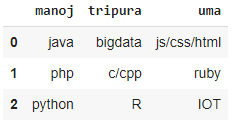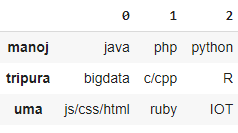# Python – Convert dict of list to Pandas dataframe

• Last Updated : 28 Nov, 2021

In this article, we will discuss how to convert a dictionary of lists to a pandas dataframe.

## Method 1: Using DataFrame.from_dict()

We will use the from_dict method. This method will construct DataFrame from dict of array-like or dicts.

Syntax:

`pandas.DataFrame.from_dict(dictionary)`

where dictionary is the input dictionary

Example: Program to take student dictionary as input and display subjects data then store in pandas dataframe

## Python3

 `# import pandas module``import` `pandas as pd`` ` ` ` `# create a dictionary for three subjects with list``# of three subjects for each student``data ``=` `{``    ``'manoj'``: [``"java"``, ``"php"``, ``"python"``],``    ``'tripura'``: [``"bigdata"``, ``"c/cpp"``, ``"R"``],``    ``'uma'``: [``"js/css/html"``, ``"ruby"``, ``"IOT"``]``}`` ` `# convert to dataframe using from_dict method``pd.DataFrame.from_dict(data)`

Output:Suppose if we want to get the dataframe with keys as row names then we have to use the orient parameter

Syntax:

`pd.DataFrame.from_dict(data,orient='index')`

Example:

## Python3

 `# import pandas module``import` `pandas as pd`` ` ` ` `# create a dictionary for three subjects with list``# of three subjects for each student``data ``=` `{``    ``'manoj'``: [``"java"``, ``"php"``, ``"python"``],``    ``'tripura'``: [``"bigdata"``, ``"c/cpp"``, ``"R"``],``    ``'uma'``: [``"js/css/html"``, ``"ruby"``, ``"IOT"``]``}`` ` `# convert to dataframe using from_dict method``# with orient``pd.DataFrame.from_dict(data, orient``=``'index'``)`

Output:## Method 2: Using pd.Series()

Here we are using Series data structure inside the dataframe method by using the items() method

Syntax:

`pd.DataFrame({ key: pd.Series(val) for key, val in dictionary.items() })`

where

• dictionary.items() is the method to get items from the dictionary
• pd.Series(val) will get series of values from the items() method

Example:

## Python3

 `# import pandas module``import` `pandas as pd`` ` `# create a dictionary for three subjects with list``# of three subjects for each student``data ``=` `{``    ``'manoj'``: [``"java"``, ``"php"``, ``"python"``],``    ``'tripura'``: [``"bigdata"``, ``"c/cpp"``, ``"R"``],``    ``'uma'``: [``"js/css/html"``, ``"ruby"``, ``"IOT"``]``}`` ` `# convert to dataframe using series with items() method``pd.DataFrame({key: pd.Series(val) ``for` `key, val ``in` `data.items()})`

Output:My Personal Notes arrow_drop_up